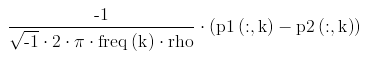# MathViz: Math expression code visualizer

### Introduction

I created an online tool called MathViz that can help you understand and debug math code written in the syntax of popular computer programming languages like MATLAB, C, Java, Python, and R. It comes in handy when you're reading other people's code, especially when it contains nested parentheses, fractions, and exponents.

For example, this line of MATLAB code is pretty hard to understand:

```(-1./(sqrt(-1)*2*pi*freq(k)*rho)).*(p1(:,k) - p2(:,k));
```

This is what it looks like when you visualize it using MathViz:It's much easier to debug math expressions when visualized in its natural form rather than as plaintext code!

### Start using MathViz

Start using MathViz right now!

### Implementation

See the source code on GitHub.

MathViz translates code written in MATLAB/C-like syntax to mathematical expressions specified in LaTeX syntax. I used PLY to generate the lexer and parser in Python and then manually wrote a few optimization passes to beautify rendering of fractions and eliminate redundant parentheses from the LaTeX output. I then added a web form interface and rendered equations using the CodeCogs online LaTeX rendering engine.